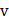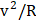# A disc is performing pure rolling on a smooth stationery surface with constant angular velocity as shown in figure. At any instant, for the lower most point of the disca) Velocity is, acceleration is zero b) Velocity is zero, acceleration is zero c) Velocity is, acceleration isd) Velocity is zero, acceleration is## Question ID - 100499 :- A disc is performing pure rolling on a smooth stationery surface with constant angular velocity as shown in figure. At any instant, for the lower most point of the disca) Velocity is, acceleration is zero b) Velocity is zero, acceleration is zero c) Velocity is, acceleration isd) Velocity is zero, acceleration is3537

(d)

As the disc is in combined rotation and translation, each point has a tangential velocity and a linear velocity in the forward directionFrom the figure,(for the lowest point)=and acceleration(Since the linear speed is constant)

Next Question :
 A solid cylinder is placed on the end of an inclined plane. It is found that the plane can be tipped at an anglebefore the cylinder starts to slide. When the cylinder turn on its sides and is allowed to roll, it is found that the steepest angle at which the ctlinder performs pure rolling is. The ratio tantanis a) 3 b)c) 1 d)